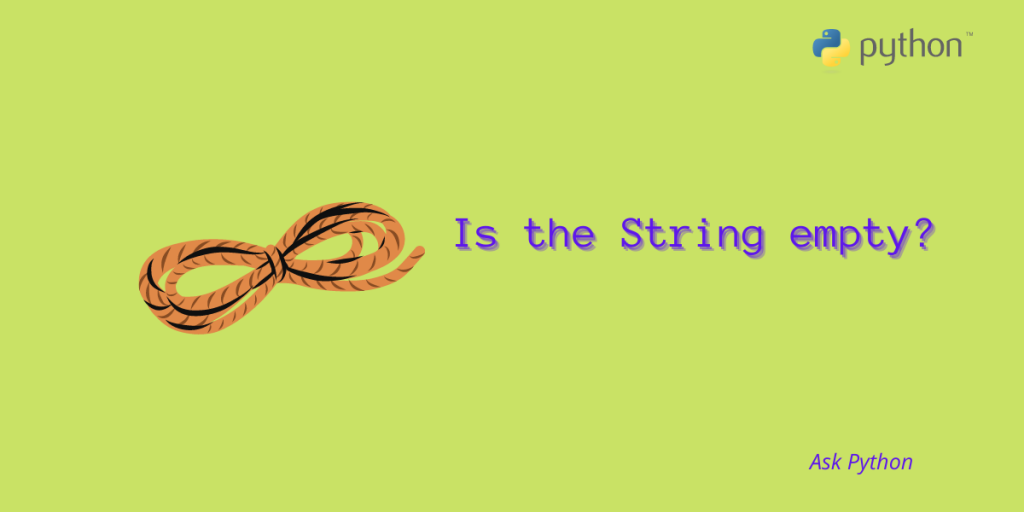# 4 Methods to Check Whether a String Is Empty in PythonThe title sounds a bit odd right as one might think we can simply check whether spring is empty or not with the help of the len() or not operator. But we need to remember here that it will space as a character in the string and will show the string as a  non-empty string. In this article, we will learn the methods we can use to check whether a string is empty or not. Let’s begin!

## Methods to Check Whether a String Is Empty in Python

Let’s look at 4 different methods to check if a string is empty or not in Python. We’ll explore each method with an example and show the output to help you perform the same on your own.

### 1. Using NOT operator

This method considers a string with spaces as a non-empty string. It counts the space in the string as a character. We should know that string with space is an empty string and has a non-zero size but this method ignores that fact.

For example

```str1 = ""
str2 = "  "

if(not str1):
print ("Yes the string is empty")
else :
print ("No the string is not empty")

if(not str2):
print ("Yes the string is empty")
else :
print ("No the string is not empty"
```

Output:

```Yes the string is empty
No the string is not empty
```

You can see it printed the string with space in it as a non-empty string.

### 2. Using len() function

Like the not operator, this also considers a string with space as a non-empty string. This method checks for non-empty zero-length strings.

For example :

```str1 = ""
str2 = "  "

if(len(str1) == 0):
print ("Yes the string is empty ")
else :
print ("No the string is not empty")

if(len(str2) == 0):
print ("Yes the strinf is empty")
else :
print ("No the string is not empty")
```

Output:

```Yes the string is empty
No the string is not empty
```

### 3. Using the not+str.strip () method

This method does not ignore the fact of an empty+non-zero-length string. Therefore, this method can serve the purpose of checking for an empty zero-length string. It looks for an empty, non-zero-length string.

For example:

```str1 = ""
str2 = "  "

if(not (str1 and str1.strip())):
print ("Yes the string is empty")
else :
print ("No the string is not empty")

if(not(str2 and str2.strip())):
print ("Yes the string is empty")
else :
print ("No the string is not empty")
```

Output:

```Yes the string is empty
Yes the string is empty
```

### 4. Using not str.isspace method

This method is similar to the above method. This method is considered to be more robust as it performs the strip operation which takes on the computational liabilities if the string contains a large number of spaces.

```str1 = ""
str2 = "  "

if(not (str1 and not str1.isspace())):
print ("Yes the string is empty")
else :
print ("No the string is not empty")

if(not (str2 and not str2.isspace())):
print ("Yes the string is empty")
else :
print ("No the string is not empty")
```

Output:

```Yes the string is empty
Yes the string is empty
```

## Conclusion

So in this article, we learned quite a lot of different methods through which we can check for an empty string. Although each method has its own drawbacks, you can use them according to your suitability.